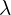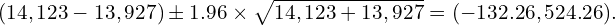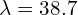# Hypothesis Testing When We Have All Data

##### Background

Classical statistical theory is built on the idea of working with a sample of data from a given population of interest. The software packages we use as data scientists compute confidence intervals to reflect precisely this – we observe only a small part of that population.

In modern times, however, we often work with all data points, and not just random samples. Examples abound, especially in the tech industry. Companies store all sales and website activity, the FBI records all homicides, and school records contain information on all students. Furthermore, this is especially relevant in B2B settings where the number of customers or transactions is often limited.

How can we interpret confidence intervals when we work with such datasets? More generally, how do we think about uncertainty in these settings? We know exactly how many items are sold or how many homicides occur each year; nothing is uncertain about that.

The short answer is that the confidence intervals in this setting have a fundamentally different interpretation – one reflecting parameters of an underlying metaphorical population. Let me explain.

##### Digging a Bit Deeper

Let’s focus on a specific example – homicides in the US. According to the Crime in the US report published by the FBI, in 2018, there were 14,123 homicides, and for 2019 this number was 13,927. This is a decrease of 196 cases, or roughly equivalent to a 1.4% drop.

Mother nature and the world around us are incredibly complex, so numbers around us can go up and down for no obvious reason. So, does this drop reflect a real change in the underlying crime rate?

To answer this question, it is helpful to model annual homicides as coming from a Poisson distribution from a figurative population of alternative US histories. This distribution has a meanequal to the hypothetical true underlying homicide rate. We want to know whetherchanged from 2018 to 2019. (See my earlier post on determining statistical significance between two quantities.)

It turns out that the confidence interval for the change in this underlying homicide rate is:This interval clearly contains 0, so we cannot conclude that there was a real drop in the crime rate between 2018 and 2019. In other words, the 1.4% drop in homicides between 2018 and 2019 was within the range consistent with the noise in our world. It should not be confused with increased underlying safety in the US.

##### One More Thing

This is not all. This type of thinking is also helpful in a slightly different context. Let’s focus on 2018, when there were 14,123 homicides in the US, corresponding to an average daily rate of about 38.7 cases.

Imagine someone asked us to calculate the probability that there would be less than 25 cases on a given day, but no such day took place in 2018. It would still be naïve to conclude that the probability of this event was zero.

We can look at the left tail of the Poisson distribution with meanto answer this question:

ppois(25, lambda=38.7)

This gives us a 1.27% probability of such an event, suggesting that, on average, there should be about 4.6 such days per year. Data would not help answer this question.

##### Bottom Line
• Working with all data eliminates the uncertainty that usually arises in random samples.
• However, confidence intervals in such settings are still meaningful – they represent uncertainty associated with the underlying parameters of a metaphorical population.